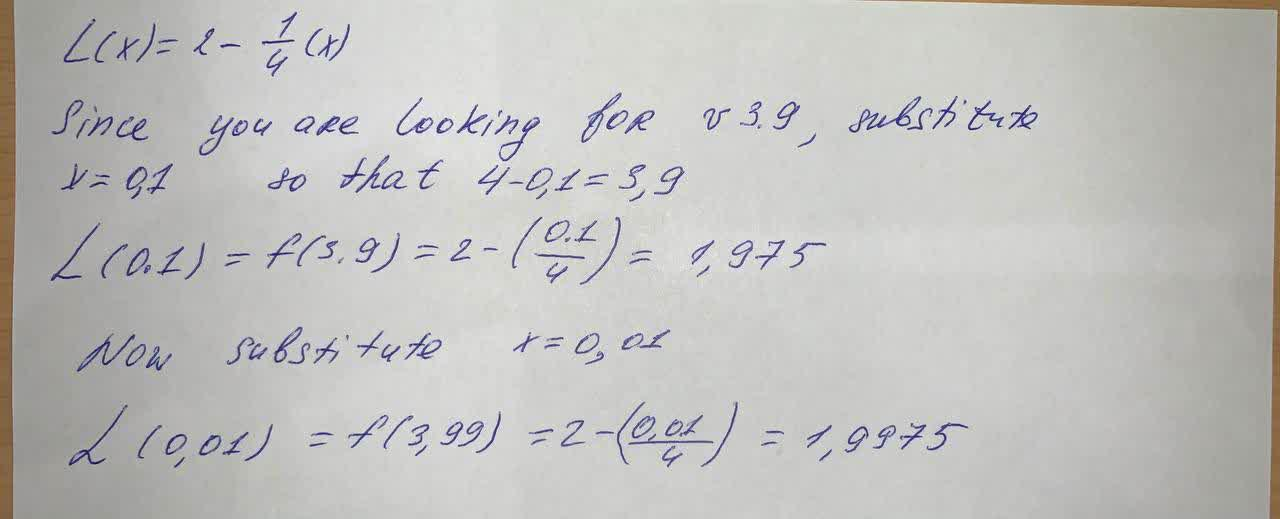# Find the linear approximation of the function f(x)=\sqrt{4-x} at a=0tabita57i 2021-08-20 Answered
Find the linear approximation of the function $$\displaystyle{f{{\left({x}\right)}}}=\sqrt{{{4}-{x}}}$$ at a=0
Use L(x) to approximate the numbers sqrt (3.9) and sqrt (3.99) Round to four decimal places

• Questions are typically answered in as fast as 30 minutes

### Plainmath recommends

• Get a detailed answer even on the hardest topics.
• Ask an expert for a step-by-step guidance to learn to do it yourself.Laith Petty

Given the function: